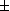# Graphic multiplication --- Introduction ---

Graphic multiplication is an exercise concerning the graphical recognition of the multiplication of functions of one real variable. The server will give you the graph of two functions $f\left(x\right)$ and g(x), whose expressions will be hidden. You will then be presented a certain number of other graphs, corresponding to functions such as $f\left(x\right)g\left(x\right)$, $f\left(-x\right)g\left(x\right)$, $f\left(x\right)g\left(x+1\right)$, etc (see the menu below). And you should locate the one which corresponds to one which will be precised in a question. To give the reply, you can simply click on the graph which you believe to be the good one.

The exercise can be configured by the following parameters.

• Type of the function to recognize:
1. xf (x), xg(x), f (x)g(x) ;
2. f 2(x), g2(x), f (x)g(x) ;
3. f (x)g(x) ;
4. f (x1)g(x1) ;
5. f (x1)g(x1), f (x)g(x)1 ;
6.f 2(x),g2(x),f (x)g(x) ;
7.xf (x),xg(x),f (x)g(x) ;
8.f (x)g(x) ;
9. xf (x), xg(x), f (x)g(x) ;
10. f (x)g(x), f (x)+g(x) ;
• Number of questions in one session : , , , , , , , (The score is attributed only at the end of a session.)
• or you can be asked to recognize each presented curve.

Other exercises on: graphic   Functions   Multiplication

In order to access WIMS services, you need a browser supporting forms. In order to test the browser you are using, please type the word wims here: and press Enter''.